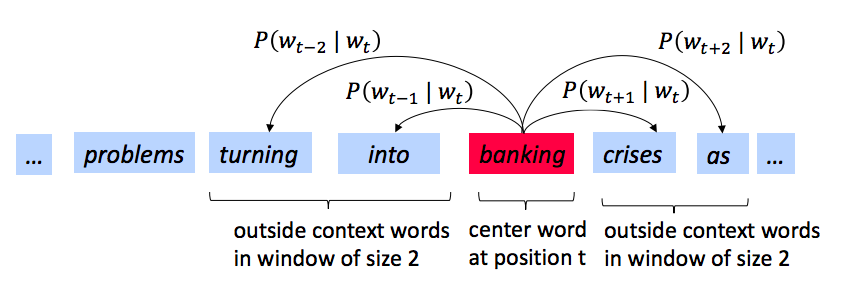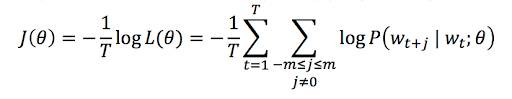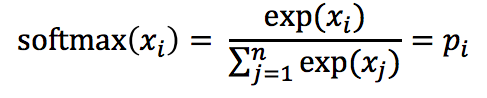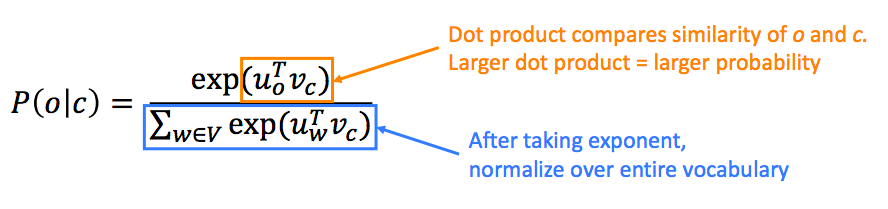Suivez votre progression
Durée estimée

# Get Started with Word2Vec

## Learning Objectives

After completing this unit, you’ll be able to:
• Describe how Word2Vec works.
• Explain the objective function Word2Vec uses.

Distributed representation gives us a way to teach computers human language and the meaning of words. But how do we actually apply it? Be prepared—we’re going to look at a lot of math in this unit!

## What Is Word2Vec?

Word2vec is an algorithm that helps you build distributed representations automatically. You feed it a large volume of text, and tell it what your fixed vocabulary should be. The algorithm then represents every word in your fixed vocabulary as a vector. Word2Vec identifies a center word (c) and its context or outside words (o). Then, it uses the similarity of the word vectors for c and o to calculate the probability of o, given c. Finally, the tool optimizes the vectors by maximizing this probability.

That’s a lot to understand. Fear not! They main thing to know here is that Word2vec has a programmatic way to calculate how often words appear next to one another. This concept is key to determining word similarity and meaning through distributed representation.

## The Objective Function

To train the model, we need an objective function that maximizes the probability of finding the words arranged as they are in the text you give it.#### Note

Remember from the previous module, an objective function (or loss function) is an equation that identifies the error between the expected result and the result your machine came up with. In this case, we want to give the machine a way to constantly learn and update the similarity between words.

So how can we go from individual probability calculations to a single equation for the entire model?

Let’s look at an example snippet of a sentence:… problems turning into banking crises as ...

We want to calculate the probability of all the outside words (o) for the word “banking” (c).To start, let’s refer to “banking” at the current position in the text (t), our center word (c) is “banking.” To make calculating the probability easier, we refer to this center word (c) as wt. Assuming we’re using a window size of 2, our context words are the words at positions t-2 (“turning”), t-1 (“into”), t+1(“crises”), and t+2 (“as”). Written another way, those are wt-2, wt-1, wt+1, and wt+2.

Now, you can express the probability of o, given c as P(o | c). So at position t-2, the probability would be P(wt-2 | wt ), and so on.

From these individual probabilities, you can write a single equation for the probability over all the data.Here’s a handy glossary for this equation:
• L—Likelihood
• θ— All the variables we can optimize to change the likelihood. In this case, that means theta is all of the word vectors we are creating and modifying, or the parameters of our model.
• t—The current position in the input text
• T—The final position in the input text
• m—The window size (how many context words we are including before and after the current center word).
• j—The index of the current context word, as it relates to the current center word. So if j = 1, we are looking at the word after the center word, if j = -1, we are looking at the word before the center word, and so on.
• wt-2—The current center word (the word at position t in the input text)

Let’s walk through the equation. It reads, “The (1) likelihood, given theta is equal to the (2) product from t=1 to T (the product at every position t throughout the body of input text) of the (3) product for each context word at that position, with a window size of m, of the (4) probability of each context word, given the current center word and the current word vectors.”

That’s some heavy duty math, but it’s important to know and have as reference as we put it to practice later in this module. Let’s simplify this even further to drive home the context. To find (1) the likelihood of having all the words arranged as they are in the input text, (2, 3) go through the entire input text, and add up (4) the probability of finding each context word near each center word, given the current word vectors.

Now that we understand how to calculate probability, we’re ready to build our objective function. The objective function comes up again and again in NLP, so this isn’t the only time you’ll see this.

We want to maximize the likelihood of finding the center words in their actual contexts, but in deep learning, we usually use an objective function that we can minimize. Why? Simply, it makes the math easier.

We could just add a negative sign and minimize the negative likelihood, but using the negative log likelihood makes it easier to take the derivative of the loss function later. The negative log likelihood of this particular arrangement of words serves as our objective function.For more about how we get from the previous equation to this one, check out this guide to Implementing word2vec in PyTorch.

## Calculating Probability

Now we’ve figured out how to create an objective function for optimizing the word vectors. You might have noticed, that we still haven’t tackled the probability calculation for individual center words! There are a few ways you can calculate the probability of finding a context word near a given center word. For the purposes of this unit, we’ll talk about a method called naive softmax. It’s not the best way to calculate probability, so it isn’t generally used in practice. However it’s a good introduction to the basic concepts.

The softmax function is a function that maps a set of arbitrary values (represented below as xi) to a probability distribution (represented below as pi).The probability distribution that softmax returns is a set with the same number of elements as the input set. Each element of this probability distribution has a value between zero and one, and the values of these elements add up to one. The softmax function magnifies the importance of the largest values in a set and minimizes the importance of the smaller values.

When we calculate the probability of o, given c, we use two vectors for each word. One vector (vw) is the vector for word w when it is the center word. The other vector (uw) is the vector for the word when it is a context word.

Because we have two vectors for each word, we can use the dot product of uo (the vector for o as a context word) and vc (the vector for c as a center word) to determine how similar o and c are. The larger the dot product, the more similar the two vectors are.#### Note

The dot product of two vectors is the sum of the products of the elements of those vectors. So, if you have these two vectors:

• v = [a,b,c]
• u = [d,e,f]
Then the dot product of v and u = ad + be + cf. Mathematically, you can denote the dot product of v and u as vTu.

This means we can find the probability of o, given c, using softmax and the dot product.:If you’re feeling bogged down by all the math, don’t worry too much. Again, these concepts are important to review and have for reference. We’ll put it all to work in the next unit.